# SBI CLERK PRELIMS – APTITUDE – 15

Dear Banking Aspirants,

The SBI CLERK Prelims Exam 2018 is one of the important exam and we know you would be searching for the correct planner to devise your strategy. Since the exam pattern is changed , you would have been puzzled about how to study for the exam and make your preparations in the right way. Don’t fret and prepare as you wish but go in the right direction.

The Preliminary Examination of the SBI CLERK 2018 will happen in March/April , so you have to gear up your preparations in the right way to score more in the exams. So attend Daily Quizzes which will enrich your preparations and study and learn new topics which will help you for the exams.

Our Team will be providing the Daily Quizzes on various topics which were based on the previous exams and new pattern questions. This will help you to crack the exam and to achieve your goal. So attend and take the best result which could guide you to find the key of your dreams.SBI Clerk Prelims 2018 Study Planner Day – 15

Section : Quantitative Aptitude

Topic : Percentages – II

Time: 20 Minutes

1) The sum of 45% of a number and 20% of the same number is 4056. What is 85% of that number?

a) 5043

b) 5430

c) 5034

d) 5340

e) 5540

2) The sum of 15% of a positive number and 10% of the same number is 70. What is twice of that number?

a) 440

b) 280

c) 560

d) 140

e) None of these

3) If a numerator of a fraction is increased by 400% and the denominator is increased by 500%, the resultant fraction is 10/21. What was the original fraction?

a) 5/12

b) 8/13

c) 17/14

d) 4/7

e) None of these

4) 10% of 20% of a number is 102. What is the number?

a) 10200

b) 10460

c) 10540

d) 10720

e) 10860

5) The difference between 58% of a number and 39% of the same number is 247. What is 62% of that number?

a) 1300

b) 806

c) 754

d) 1170

e) None of these

6) There are 1240 employees in an organization. Out of which 25% got promoted. How many such employees are there who got promotion?

a) 398

b) 345

c) 310

d) 372

e) None of these

7) Bina’s monthly income is 90% of Anita’s monthly income. The total of both their monthly incomes is Mr. Sen’s monthly income. Mr. Sen’s annual income is Rs. 775200. What is Bina’s monthly income? (In some cases, annual income is asked and, in some case, monthly income is asked)

a) Rs. 34000

b) Rs. 36000

c) Rs. 30600

d) Rs. 30000

e) None of these

8) The product of 5% of a positive number and 3% of the same number is 504.6. What is half of that number?

a) 290

b) 340

c) 680

d) 580

e) None of these

9) Three-fourth of a number is equal to 60% of another number. What is the difference between the numbers?

a) 18

b) 32

c) 24

d) Cannot be determined

e) None of these

10) Half of a number is equal to 65% of another number. What is the ratio between the first number and the second number respectively?

a) 10:13

b) 8:13

c) 13:8

d) 13:10

e) None of these

11) Rajesh decided to donate 16% of his salary on the day of donation he changed his mind and donated Rs. 4320 which was three-fifths of what he had decided earlier. How much is the salary of Rajesh?

a) Rs. 45000

b) Rs. 45800

c) Rs. 46000

d) Rs. 46400

e) None of these

12) Deepa decided to donate 8% of her salary to an orphanage. On the day of donation, she changed her mind and donated Rs. 2240 which was 80% of what she had decided earlier. How much is Deepa’s salary?

a) Rs. 36000

b) Rs. 42000

c) Rs. 35000

d) Rs. 45000

e) Rs. 45000

13) A student has to secure 36% marks to pass. He gets 150 marks and fails by 30 marks. The maximum marks are

a) 540

b) 600

c) 500

d) 620

e) None of these

14) Mr. Natwarlal gave 50% of the money he had to his wife, and the remaining amount he distributed equally among his three sons. If each son got Rs. 3250, what was the total amount he had?

a) Rs. 13000

b) Rs. 19500

c) Rs. 16250

d) Cannot be determined

e) None of these

15) In an examination Shalini scores a total of 436 marks out of 625. What is her approximate percentage in the examination?

a) 83

b) 65

c) 82

d) 78

e) 70

16) 25% of 50% of a number is 385. What is the number?

a) 3050

b) 3100

c) 3004

d) 3080

e) None of these

17) Bhoomi invests Rs. 3675, which is 14% of her monthly income in mutual funds. What is her monthly income?

a) Rs. 23700

b) Rs. 28450

c) Rs. 26250

d) Rs. 25950

e) None of these

18) Anish spends 25% of his salary on house rent, 5% on food, 15% on travel. 10% on clothes and the remaining amount of Rs. 22500 is saved. What is Anish’s salary?

a) Rs. 40000

b) Rs. 40500

c) Rs. 45500

d) Rs. 50000

e) None of these

19) Twenty-seven percent of Arun’s monthly salary is equal to Ruju’s monthly salary. If Arun’s monthly salary is Rs. 25000, then what is Ruju’s annual salary?

a) Rs. 87000

b) Rs. 810000

c) Rs. 111000

d) Rs. 57000

e) None of these

20) The present ages of Aunil and Anil are in the ratio of 7:8 respectively. If four years ago the ratio of their ages was 5:6 respectively, what is Anil’s present age in years?

a) 16

b) 14

c) 10

d) 12

e) None of these

Solutions for the Above Questions :

1. (D)

45% of (x) + 25% of (x) = 4056

X = 6240

85% of (6240) = 5304

1. (C)

Sum of numbers = 15% of (x) + 10% of (x) = 70

X = 280

Twice the number = 2 × 280 = 560

1. (D)

Numerator of fraction increased 400%

So, 500% of (x)

Denominator increased 500%, so 600% of (y)

Resultant =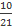500% of (x) ÷ 600% of (y) = 10 ÷ 21

Original fraction =1. (A)

10% of 20% of ½ of a number is 102.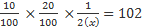1. (B)

58% of x-39% of x=247

-> 0.58x-0.39x=247->x=1300

Required Answer = 0.67 * 1300 =806

1. (C)

According to question,

1240*25/100=310

1. (C)

Bina’s monthly income (B) = 90/100 Anitha’s monthly income (A)

A+B = Sen’s monthly income(S)

Sen’s monthly income =775200/12=Rs.64600

According to question

A+0.9A=64600->1.9A=64600-> A = Rs.34000

Therefore, Bina’s Monthly income (B) = 64600-34000= Rs.30600

1. (A)

According to the question1. (D)

According to the question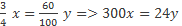So, we cannot be determined

1. (D)

According to the question: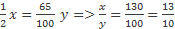1. (A)

Rajesh salary =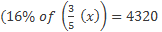X = 45000

1. (C)

Deepa’s salary = 8% of (80% (x)) = 2240

X= 350

1. (C)

Student has to secure 36% to pass

Secured – 150 marks

Failed by- 30 marks

Maximum marks are = 36% of (x) = 180 => x = 500

1. (B)

Remaining amount = 50%

Sharing between 3 sons so we get = 50% of (x) = 3 × 3250 => x = 19500

1. (E)

Shalini got 436 out of 625

Percentage in an examination =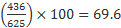1. (D)

25% of 50 % (x) = 385

X = 3080

1. (C)

Boomi’s monthly income = 14% of (x) = 3675 => x = 26250

1. (D)

Anish spent his salary = 55%

Remaining salary = 45%

45% of (x) = 22500

X = 50000

1. (E)

27% (A) = R

R’s monthly salary =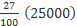R’s monthly salary = 6750

R’s yearly salary = 6750 × 12 = 81000

1. (A)

Present ages of Aunil and Anil =7: 8

4 years ago, = 5: 6

7x-4/8x-4 = 5/6

X = 2

Anil’s present age = 16

Aspirants can also check the other topics from the SBI CLERK STUDY PLANNER from the link given below. DO bookmark the given link as all the posts will be updated in that page and aspirants can check the daily updates from this page or from the Homepage of Bankersdaily.